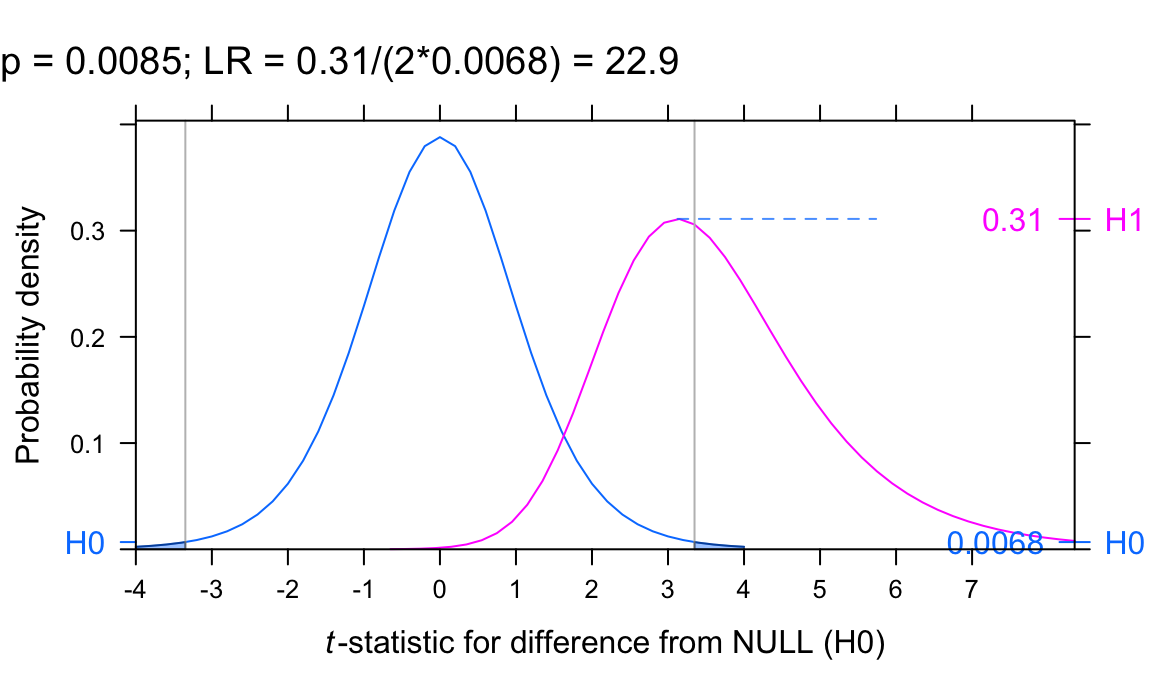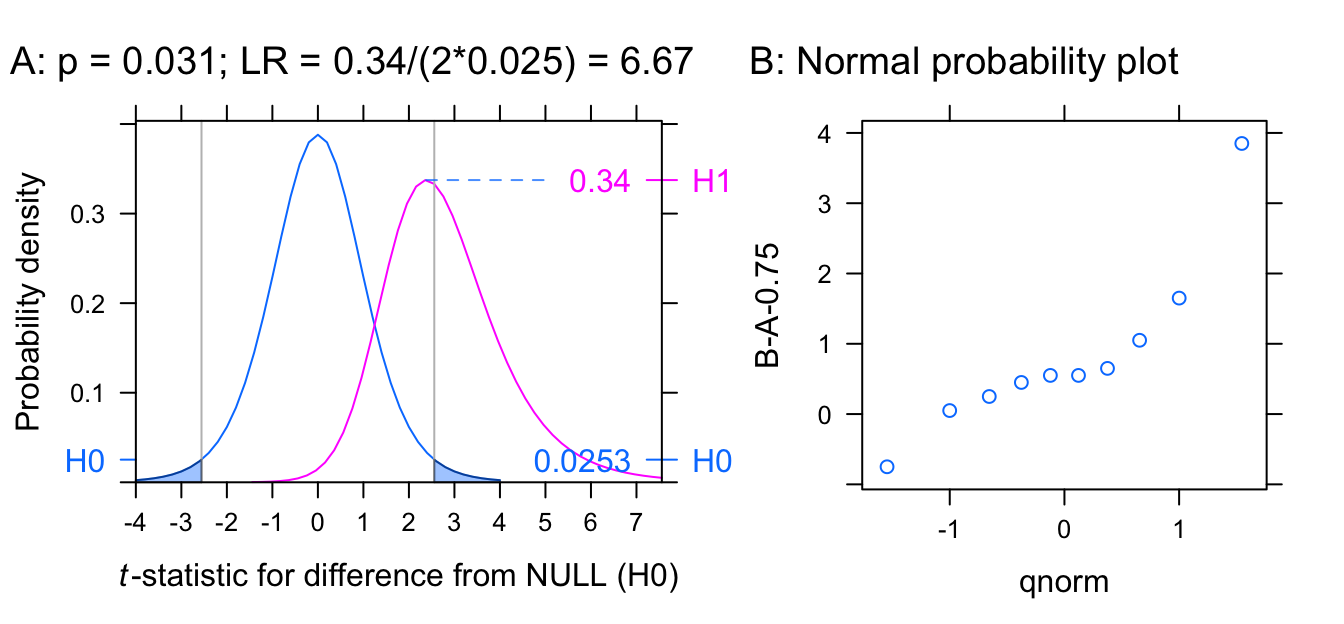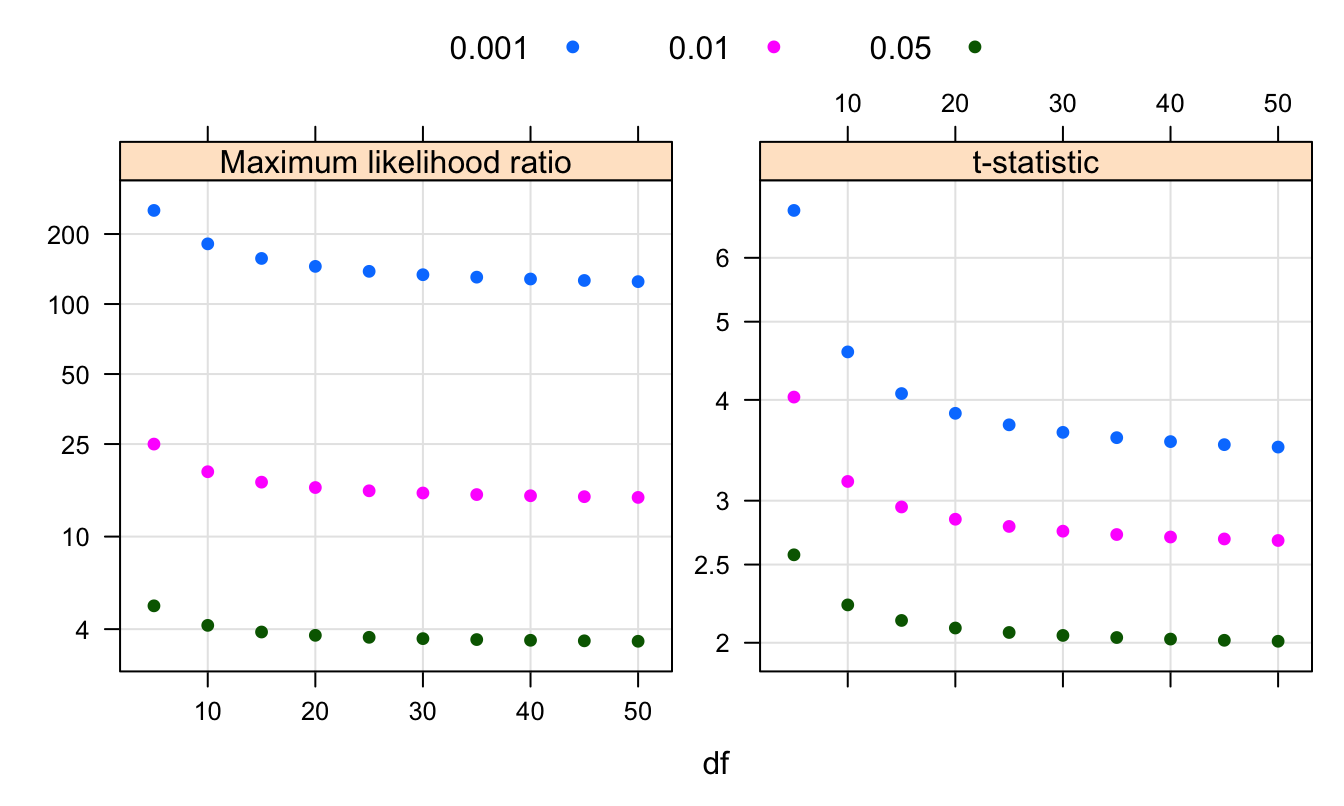## Introduction

Data from an experiment that compares results from a treatments with a baseline provides a relatively simple setting in which to probe the interpretation that should be placed on a given $$p$$-value. Even in this ‘simple’ setting, the issues that arise for the interpretation of a $$p$$-value, and its implication for the credence that should be given to a claimed difference, are non-trivial.

$$P$$-values are calculated conditional on the null hypothesis being true. In order to obtain a probability that the null hypothesis is true, they must be supplemented with other information. $$P$$-values do not answer the questions that are likely to be of immediate interest. Berkson (1942) makes the point succinctly:

If an event has occurred, the definitive question is not, Is this an event which would be rare if the null hypothesis is true?’ but ‘Is there an alternative hypothesis under which the event would be relatively frequent?’

Of even more interest, in many contexts, is an assessment of the false positive risk, i.e., of the probability that, having accepted the alternative hypothesis, it is in fact false. This requires an assessment of the prior probability that the null is true. The best one can do, often, is to check how the false positive risk may vary with values of the prior probability that fall within a range that is judged plausible.

In the calculation of a $$p$$-value, there is regard both to the value of a statistic that has been calculated from the observed data, and to more extreme values. This feature attracted the criticism, in Jeffreys (1939), that “a hypothesis which may be true may be rejected because it has not predicted observable results which have not occurred.” The use of likelihoods, which depend only on the actual observed data and have better theoretical properties, avoids this criticism. A likelihood is a more nuanced starting point than a $$p$$-value for showing how the false positive risk varies with the prior probability.

## Introduction

Data from an experiment that compares results from a treatments with a baseline provides a relatively simple setting in which to probe the interpretation that should be placed on a given $$p$$-value. Even in this ‘simple’ setting, the issues that arise for the interpretation of a $$p$$-value, and its implication for the credence that should be given to a claimed difference, are non-trivial.

$$P$$-values are calculated conditional on the null hypothesis being true. In order to obtain a probability that the null hypothesis is true, they must be supplemented with other information. $$P$$-values do not answer the questions that are likely to be of immediate interest. Berkson (1942) makes the point succinctly:

If an event has occurred, the definitive question is not, Is this an event which would be rare if the null hypothesis is true?’ but ‘Is there an alternative hypothesis under which the event would be relatively frequent?’

Of even more interest, in many contexts, is an assessment of the false positive risk, i.e., of the probability that, having accepted the alternative hypothesis, it is in fact false. This requires an assessment of the prior probability that the null is true. The best one can do, often, is to check how the false positive risk may vary with values of the prior probability that fall within a range that is judged plausible.

In the calculation of a $$p$$-value, there is regard both to the value of a statistic that has been calculated from the observed data, and to more extreme values. This feature attracted the criticism, in Jeffreys (1939), that “a hypothesis which may be true may be rejected because it has not predicted observable results which have not occurred.” The use of likelihoods, which depend only on the actual observed data and have better theoretical properties, avoids this criticism. A likelihood is a more nuanced starting point than a $$p$$-value for showing how the false positive risk varies with the prior probability.

# 1 Making sense of $$p$$-values

The Null Hypothesis Significance Testing (NHST) approach to statistical decision making sets up a choice between a null hypothesis, commonly written H$$_0$$, and alternative H$$_1$$, with the calculated $$p$$-value used to decide whether H$$_0$$ should be rejected in favour of H$$_1$$. In a medical context, a treatment of interest may be compared with a placebo.

Such a binary choice is not always appropriate. There are many circumstances where it makes more sense to treat the problem as one of estimation, with the estimate accompanied with a measure of accuracy.

## 1.1 Examples that illustrate key points

### Wear comparison for two different shoe materials

A simple example will serve as a starting point for discussion. The dataset compares, for each of ten boys, the wear on two different shoe materials. Materials A and B were assigned at random to feet — one to the left foot, and the other to the right. The measurements of wear, and the differences for each boy, were:

wear <- with(MASS::shoes, rbind(A,B,d=B-A))
colnames(wear) <- rep("",10)
wear

A 13.2 8.2 10.9 14.3 10.7  6.6 9.5 10.8 8.8 13.3
B 14.0 8.8 11.2 14.2 11.8  6.4 9.8 11.3 9.3 13.6
d  0.8 0.6  0.3 -0.1  1.1 -0.2 0.3  0.5 0.5  0.3

The differences are then used to calculate a $$t$$-statistic, on the basis of which, a statistical test is performed that is designed to help in choosing between the alternatives:

• H0: $$\mu$$ = 0 (the NULL hypothesis)
• H1: $$\mu \neq 0$$ (a 2-sided test) or $$\mu > 0$$ (a 1-sided test), for the alternative.

The $$p$$-value is calculated, assuming that the differences $$d_i, i=1, 2, \ldots 10$$ have been independently drawn from the same normal distribution. The statistic $$\sqrt{n} \bar{d}/s$$, where $$\bar{d}$$ is the mean of the $$d_i$$, and $$s$$ is the sample standard deviation, can then be treated as drawn from a $$t$$-distribution. The $$p$$-value for a 2-sided test is then, assuming H0, and as any difference might in principle go in either direction:

the probability of occurrence of values of the $$t$$-statistic $$t$$ that are greater than or equal to $$\sqrt{n} \bar{d}/s$$ in magnitude

It is, also, the probability that:

a $$p$$-value calculated in this way will, under the same NULL hypothesis assumptions, be less than or equal to the observed $$p$$.

These definitions may seem, if serious attention is paid to them, contorted and unhelpful. The discussion that follows will, as well as commenting on common misunderstandings, examine perspectives on $$p$$-values that will help explain how they can be meaningfully interpreted. Just as importantly, how should they be used?

In other words, use $$p$$-values as a screening device, to identify results that may merit further investigation. This is very different from the way that $$p$$-values have come to be used in most current scientific discourse. A $$p$$-value should be treated as a measure of change in the weight of evidence, not a measure of the absolute weight of evidence.

A researcher will want to know: ‘’Given that the value observed is $$p$$, not some smaller value, what does this imply for the conclusions that can be drawn from the experimental data?’’ Additional information, and perhaps a refining of the question, if one is the say more than that: ‘’As the $$p$$-value becomes smaller, it becomes less likely that the NULL hypothesis is true.’’

In the sequel, likelihood ratio statistics will be examined, both for the light that they shed on $$p$$-values, and as alternatives to $$p$$-values. It is necessary to consider carefully just what likelihood ratio best fits what the researcher wants to know. What is the smallest difference in means that is of practical importance?

There are two cases to consider — the one-sample case, and the two-sample case. The discussion that follows will focus on the one-sample case. This may arise in two ways. A treatment may be compared with a fixed baseline, or units in a treatment may be paired, with the differences $$d_i, i=1, 2, \ldots, n$$ used for analysis. The $$p$$-value for testing for no difference is obtained by referring the $$t$$-statistic for the mean $$\bar{d}$$ of the $$d_i$$ to a $$t$$-distribution with $$n-1$$ degrees of freedom.

### Results from a $$t$$-test – shoe wear data

The dataset is the first of two datasets with which we will work. As noted above, it compares, for each of $$n = 10$$ boys, the wear on two different shoe materials. Results from a $$t$$-test for the NULL hypothesis that the differences are a random sample from a normal distribution with mean zero gives the result:

  Mean     SD      n    SEM      t   pval     df
0.41   0.39     10   0.12    3.3 0.0085      9 

Figure 1.1 compares the density curves, under H0 and under an alternative H1 for which the mean of the $$t$$-distribution is $$\bar{d}$$. Notice that, in each panel, the curve for the alternative is more spread out than the curve for the NULL, and is slightly skewed to the right, and the mode (where the likelihood is a maximum) is slightly to the left of the mean, This is because the distance between the curves, as measured by the non-centrality parameter for the $$t$$-distribution for the alternative, is subject to sampling error.Figure 1.1: Panel A shows density curves for NULL and for the alternative, for a two-sided test with $$t$$ = 3.35, on 9 degrees of freedom, for the comparison of shoe materials (B versus A) in the dataset MASS::shoes. Vertical lines are placed at the positions that give the $$p$$-value. Panel B shows the normal probability plot for the differences in the dataset.

Likelihood ratios offer useful insights on what $$p$$-values may mean in practice. Figure 1.1 gives the maximum likelihood ratio as 22.9. In the absence of contextual information that gives an indication of the size of the difference that is of practical importance, the ratio of the maximum likelihood when the NULL is false to the likelihood when the NULL is true gives a sense of the meaning that can be placed on a $$p$$-value. If information is available on the prior probability, or if a guess can be made, it can be immediately translated into a false positive risk statistic.

Irrespective of the threshold set for finding a difference, both $$p$$ and the likelihood ratio will detect increasingly small differences from the NULL as the sample size increases. A way around this is to set a cutoff for the minimum difference of interest, and calculate the difference relative to that cutoff. It is simplest to do that for a one-sided test. The use of a cutoff will be illustrated using the second dataset.

### Soporofic drugs: comparison of effectiveness

The dataset datasets::sleep has the increase in sleeping hours, on the same set of patients, on each of the two drugs. Data, with output from a two-sided $$t$$-test, are:

sleep2 <-with(sleep, rbind(Drug1=extra[group==1], Drug2=extra[group==2],
d=extra[group==2]-extra[group==1]))
colnames(sleep2) <- rep("",10)
sleep2

Drug1 0.7 -1.6 -0.2 -1.2 -0.1 3.4 3.7 0.8 0.0 2.0
Drug2 1.9  0.8  1.1  0.1 -0.1 4.4 5.5 1.6 4.6 3.4
d     1.2  2.4  1.3  1.3  0.0 1.0 1.8 0.8 4.6 1.4
t <- t.test(-extra ~ group, data = sleep, paired=TRUE)

The $$t$$-statistic is 4.06, with $$p$$ = 0.0028. The $$p$$-value translates to a maximum likelihood ratio that equals 67.6, which suggests a very clear difference in effectiveness.

### A test that sets $$\mu$$ = 0.75 hours as the baseline

Suppose, now, that 0.75 hours difference is set as the minimum that is of interest. As we are satisfied that drug B gives a bigger increase, and we wish to check the strength of evidence for an increase that is 0.75 hours of more, a one-sided test is appropriate. Figure 1.2A shows the comparison on the densities.Figure 1.2: Panel A shows density curves for NULL and for the alternative, for a one-sided test with $$t$$ = 2.01 on 9 degrees of freedom. This is the $$t$$-statistic for the data on the effect of soporofic drugs when differences are , i.e., interest is in the strength of evidence that differences are at least 0.75 hours. A vertical line is placed at the position that gives the $$p$$-value. Panel B shows the normal probability plot for the differences.

Calculations can be done thus:

t <- t.test(-extra ~ group, data = sleep, mu=0.75,
paired=TRUE, alternative = 'greater')

The $$t$$-statistic is 2.13, with $$p$$ = 0.0308. The maximum ratio of the likelihoods, given in Figure 1.2A as 3.5, is much smaller than the value of $$p^{-1}-1$$ = 31.5.

The normal probability plot shows a clear departure from normality.  At best, the $$p$$-values give ballpark indications.

There are other ways to calculate a likelihood ratio. In principle, one might calculate the average for all values where $$\bar{d}$$ is greater than the cutoff. This, however, requires an assumed distribution for $$\bar{d}$$ under the alternative. It can never exceed the maximum value, calculated as in Figure 1.2A

## 1.2 Use of a cutoff $$\alpha$$ versus the calculated $$p$$-value

In the discussion to date, we have worked with the calculated $$p$$-value. Note the distinction between:

1. Choosing a cutoff $$\alpha$$ in advance, treating all values $$p$$ that are less than $$\alpha$$ as evidence of a real difference, and ignoring the more nuanced information provided by the actual calculated $$p$$-value.
• Under the NULL hypothesis, the probability of (falsely) finding a difference is then $$\alpha$$.
• The ratio $$(\alpha^{-1}-1):1$$, which is 19:1 for $$\alpha$$ = 0.05, has the role of a likelihood ratio.
• Here, what is prescribed is a strategy. Its consequences have to be evaluated by studying what happens when it is applied, repeatedly, over (infinitely) many results.
2. The magnitude of the individual $$p$$-value.
• Under H0, $$p$$ is uniformly distributed on the interval $$0 <= p <= 1$$.
• The individual $$p$$-value is at the upper end of the range of values of which account is taken. It lies in the middle of a range of values that extend from 2$$p$$ to 0 and which, under the NULL, occur with probability 2$$p$$.
This suggests a form of equivalence between a calculated $$p$$-value $$p$$ and $$\alpha = 2p$$.

## 1.3 Common sources of misunderstanding

Two common misinterpretations of $$p$$-values are:

• The $$p$$-value gives the probability that the NULL hypothesis is false.
• This is wrong because the $$p$$-value is calculated under the assumption that the NULL hypothesis is false. It cannot tell us the probability that the assumption under which it is calculated is correct.
• The $$p$$-value is the probability that the results occurred by chance.
• In order to calculate this, one needs to know how many of the positives are true positives.

These statements are also wrong if, in the case where a a cutoff $$\alpha$$ has been chosen in advance, $$p$$ is replaced by $$\alpha$$.

Resnick (2017) makes the point thus:

The tricky point is then, that the $$p$$-value does not show how rare the results of an experiment are. It’s how rare the results would be in the world where the null hypothesis is true. That is, it’s how rare the results would be if nothing in your experiment worked, and the difference … was due to random chance alone. The $$p$$-value quantifies this rareness.

It is important to show that the there is an alternative hypothesis under which the observed data would be relatively more likely. Likelihood ratio statistics address that comparison directly, where $$p$$-values do not. Where there is a prior judgement on the extent of difference between $$H_1$$ and $$H_1$$ that is of of practical interest, this may have implications for the choice of statistic.

### One experiment may not, on its own, be enough

Note comments from Fisher (1935), who introduced the use of $$p$$-values, on their proper use:

No isolated experiment, however significant in itself, can suffice for the experimental demonstration of any natural phenomenon; for the ‘one chance in a million’ will undoubtedly occur, with no less and no more than its appropriate frequency, however surprised we may be that it should occur to us. In order to assert that a natural phenomenon is experimentally demonstrable we need, not an isolated record, but a reliable method of procedure. In relation to the test of significance, we may say that a phenomenon is experimentally demonstrable when we know how to conduct an experiment which will rarely fail to give us a statistically significant result.

# 2 Likelihood ratio and false positive risk

## 2.1 The maximum likelihood ratio $$p$$-value equivalent

Note again that we have been dividing the maximum likelihood for the alternative by the likelihood for the NULL.Figure 2.1: Ratio of the maximum likelihood under the alternative to the likelihood under the NULL, for three different choices of $$p$$-value, for a range of sample sizes, and for a range of degrees of freedom.

Figure 2.1 gives the maximum likelihood ratio equivalents of $$p$$-values, for a range of sample sizes, for $$p$$-values that equal 0.05, 0.01, and 0.001, and for a range of degrees of freedom. The comparison is always between a point NULL (here $$\mu$$=0) and the alternative $$\mu$$ > 0. Notice that, for 6 or more degrees of freedom $$p$$ = 0.05 translates to a ratio that is less than 5.0, while it is less than 4.5 for 10 or more degrees of freedom.

What is true is that the NULL hypothesis becomes less likely as the $$p$$-value becomes smaller. Additional information is required, if we are to say just how small. The same applies where $$p$$ is replaced by a cutoff $$\alpha$$ — as $$\alpha$$ becomes smaller, the NULL hypothesis becomes less credible. The relative amount by which credibility reduces depends on the alternative that is chosen.

## 2.2 False positive risk versus p-value

What is the probability, under one or other decision strategy, that what is identified as a positive will be a false positive? False positive risk calculations require an assumption about the prior distribution.

The false positive risk can be calculated as (1-prior)/(1-prior+prior*lr), where prior = $$\pi$$ is the prior probability of the alternative H1, with 1-prior as the prior probability of H0.